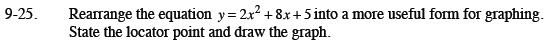Home > CCA2 > Chapter 9 > Lesson 9.1.2 > Problem9-25

9-25.

Rearrange the equation y = 2x2 + 8x + 5 into a more useful form for graphing. State the locator point and draw the graph. Homework Help ✎Complete the square.

Factor a 2 out of the first two terms.

$y=2(x^{2}+4x)+5$

Add 4 inside the ( )s which is really adding 8 because of the 2 multiplier. So, subtract 8 on the same side to keep the same value.

$y=2(x^{2}+4x+4) +5 -8$

Simplify.

$y=2(x+2)^{2}-3$